Breaking News

#### Select Language

A quadratic equation is an equation where the power on the variable is at most 2.

Quadratic equations differ from linear equations by the fact that a linear equation only has one solution, while a quadratic equation has at most two solutions. There are some special situations when a quadratic equation only has one solution.

We solve quadratic equations by factorisation, that is writing the quadratic as a product of two expressions in brackets. The follow examples, will take you through the steps required to achieve the desired results, when solving quadratic equations.

Example 1: what solutions for x are true in this quadratic equation; 2x² – 5x – 12 = 0

Step 1: Determine whether the equation has common factors, the equation has no common factors.

Step 2: Determine if the equation is in the form ax² + bx + c, and if a>0. The equation is in the required form a=2 and 2>0.

Step 3: Factorise the quadratic expression 2x² – 5x – 12, and it should have the factors of the form (2x+s)(x+v), with s and v constant to be determined. Then, the above expressions in brackets; I.e (2x+s)(x+v); should expand out to become 2x² + (s+2v)x + sv , you see that sv=-12 and s+2v = -5 ; this is a set of simultaneous equations in s and v, but it is easy to solve numerically. All the options for s and v are carried out on the image below.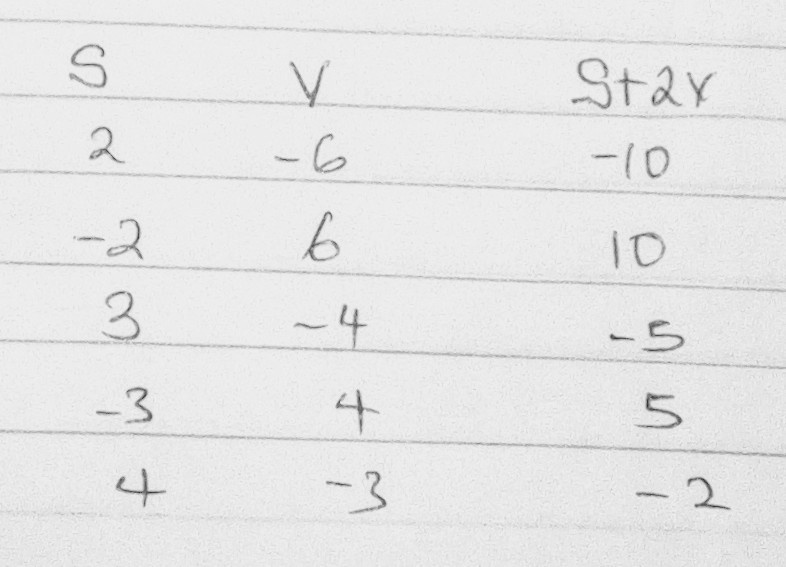from the image above, you have seen that the combinations s=3 and v=-4 gives s+2v=-5.Step 4: write the equation with its factors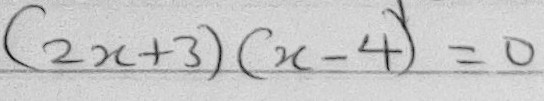Step 5: If two brackets are multiplied together to get zero, then one of the brackets must be zero, therefore solve for x in each bracket.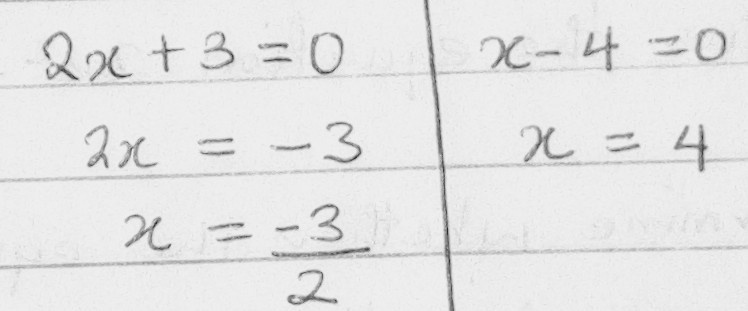Conclusion: x = -3/2, or x = 4 are the solution of the quadratic equation 2x² – 5x – 12 = 0.Example 2; what are the possible values for x; for x(x-3)=10, to become true.

Step 1: Rewrite the quadratic equation in the form of ax² + bx + c, therefore, if you remove the brackets and move all the terms to the left hand side of the equation then, x(x-3)=10 should become x² -3x-10 = 0.

Step 2: Factorise the equation x² -3x-10 = 0 , and if the equation is Factorised correctly you should get (x+2)(x-5)=0,

Step 3: Since, two brackets are multiplied together to get zero, then one of the brackets must be zero, therefore solve for x in each bracket.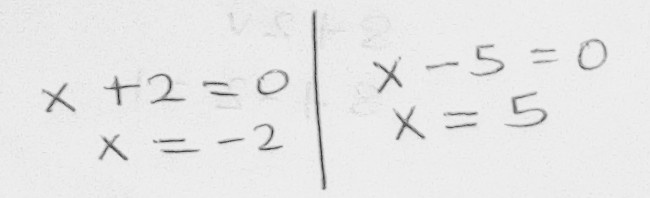Conclusion: x = -2, or x = 4 are the possible solution which makes the quadratic equation x(x-3)=10 true.

Example 4; Solve for the value of x in the quadratic equation on the image below.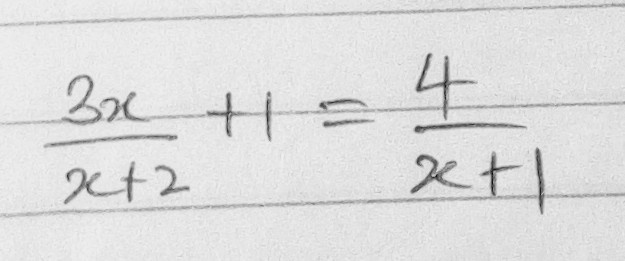Step 1: Multiply both sides of the equation by the LCM, and Put both sides over the LCM.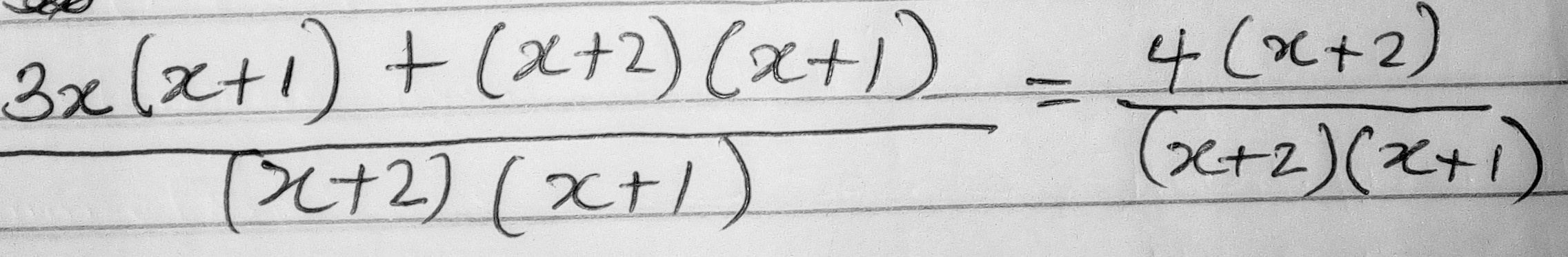Step 2: Determine the restrictions of the arrangement in the image above; Since, the denominators are the same, the numerators must be the same. Since, x ≠ -2 and x ≠ -1,Simplify the equation to the standard form ax² + bx + c.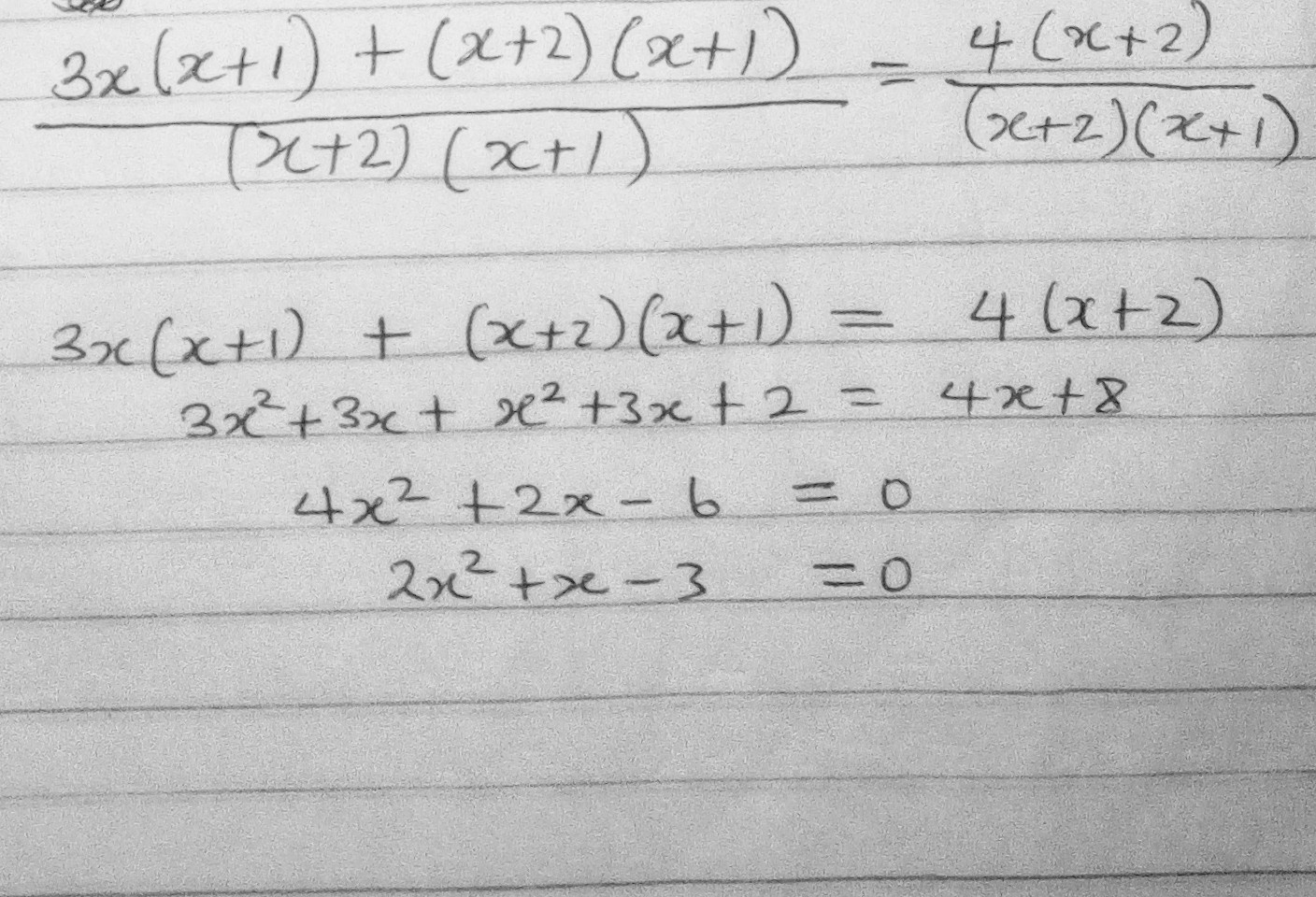Step 3: Factorise the equation 2x² +x-3 = 0 , and if the equation is Factorised correctly you should get (2x+3)(x-1)=0,

Step 4: Since both brackets, when multiplied together, will give zero, then one of the brackets must be zero, therefore solve for x in each bracket.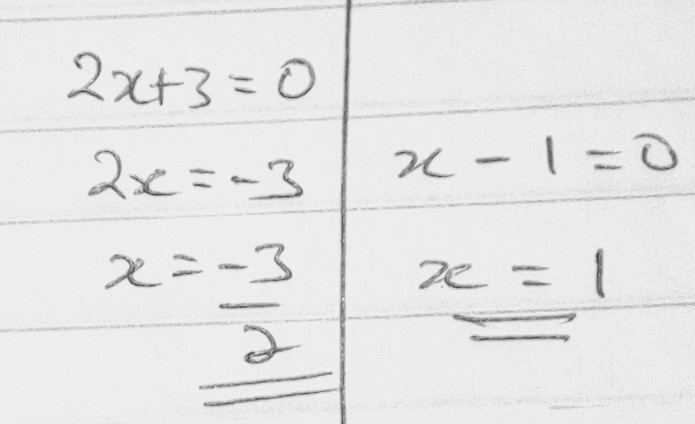Conclusion: x = -3/2, or x = 1 are the possible solution for the quadratic equation on the image belowNGstudents: For Educational Information, Local And World Breaking News

1.Aaron // __ August 12, 2020 at 8:49 AM // Reply

Thanks Man

Like

2.Anonymous // __ August 12, 2020 at 6:38 PM // Reply

I need full math’s questions and answers to 2020 waec before 17 August please

Like

3.Chinedum Augustine Obinna // __ August 14, 2020 at 4:51 PM // Reply

Nice

Like